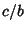If proofreaderfindsmistakes and proofreaderfindsmistakes,of which were also found by, how many mistakes were missed by bothand? Assume there are a total ofmistakes, so proofreaderfinds a Fractionof all mistakes, and also a Fractionof the mistakes found by. Assuming these fractions are the same, then solving forgivesThe number of mistakes missed by both is therefore approximatelyReferences

Pólya, G. Probabilities in Proofreading.'' Amer. Math. Monthly, 83, 42, 1976.